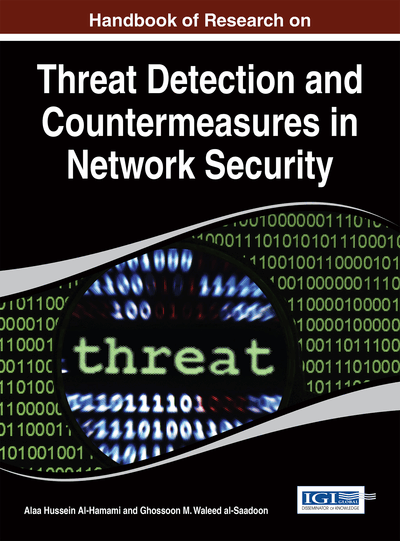# Cryptographic Hash Function: A High Level View

DOI: 10.4018/978-1-4666-6583-5.ch006

## Abstract

Cryptographic hash function verifies data integrity and sender identity or source of information. The task is accomplished by taking a variable bit patterns as an input then produces a fixed bit patterns of output. This chapter provides a detailed overview to include classification, properties, constructions, attacks, applications and an overview of a selected dedicated cryptographic hash function.
Chapter Preview
Top

## Introduction

A message digest {0,1}n is an algorithm H that uses a un-constant size message as input {0,1}* to produce a constant size output (sometimes called a digital fingerprint, imprint, hash result, hash code, hash value, or purely hash). All above alterative names were actually functions which made to play a fundamental role in modern cryptography practical applications, for example, a digital signature (Goldwasser et al, 1988), digital time stamp (Haber & Stornetta, 1991), message authentication code (or MAC) (Krawczyk et al, 1997), public key encryption (Cramer & Shoup, 2003), tamper detection of files and many more. Due to the application versatility it deserved the nickname “Swiss army knife of cryptography”.

### Background

Cryptology is the science of hiding and revealing information. This term is subdivided into two categories; cryptography and cryptanalysis. Cryptography is the science of studying the information security. Where, its opposite term is cryptanalysis, which refers to the science of violating the information security. While, cryptographer studies the techniques needed to achieve and protect the information between any communication channels, cryptanalyst studies the techniques needed to understand the meaning of secret information and attempts to break them. More precisely, Whitfield Diffie and Martin Hellman, in their seminal paper on “New Directions in Cryptography” (Diffie & Hellman, 1976), they have given birth to many concepts that are widely used these days by clearly distinguishing between privacy and authentication. Their definition of cryptography is “Cryptography is the study of mathematical systems for solving two kinds of security problems: privacy and authentication”. Where, privacy of information ensures the secrecy of the information, and authenticity proves sender’s identity. Another new definition proposed by Ronald Rivest (Rivest, 1991), he noted in chapter “Cryptography” in the “Handbook of Theoretical Computer Science” that cryptography is about communication in the presence of adversaries. The adversary is the enemy or opponent who aims to prevent the cryptographers of the cryptosystems to achieve their protection security goals. The adversaries can be in form of humans, computers operated or programmed by humans. The modern cryptography concept presented new directions of security measurements that should be considered when designing a cryptosystem. More precisely, modern cryptography is all about constructing and analyzing protocols that overcome adversaries’ threats that are related to information security. Where, information security falls into four measurements: data confidentiality, data integrity, authenticity and non-repudiation. To achieve the information security measurements, modern cryptography can be divided into several area of study. Cryptographic hash function is one branch of the cryptography family tree. Cryptographic hash function is a function that takes an arbitrary length as an input and produces a fixed size of an output. The viability of using keyed cryptographic hash function is to verify data integrity and sender identity or source of information. Consequently, the digital Holy Quran requires a keyed cryptographic hash function as protection method from any alteration or modification. Next sections will overview cryptographic hash functions in more details.

## Key Terms in this Chapter

Keyed Hash Functions: Accept a variable length message and a fixed length secret key as two inputs to the hash function design to produce a fixed length hash digest, H: {0,1} k ×{0,1}*?{0,1} n

One-Way Hash Function: A hash function where finding an input which hashes to a pre-specified hash digest is difficult.

Compression Function: H maps an input x of arbitrary finite bit length, to an output h(x) of fixed bit length n.

Universal One-Way Hash Function: For randomly chosen input x, key k and the function Hk, it is hard to find y = x such that Hk(x) = Hk(y).

Unkeyed Hash Functions: Accepts a variable length message as a single input and produce a fixed hash digest, H: {0,1}*?{0,1}n. It is also known as modification detection codes (MDCs).

Cryptographic Hash Function: An algorithm that takes an arbitrary length of message as an input {0, 1}* and produce a fixed length of an output called message digest {0, 1}n (sometimes called an imprint, digital fingerprint, hash code, hash result, hash value, or simply hash).

Collision Resistant Hash Function: A hash function where finding any two inputs having the same hash digest is difficult.

## Complete Chapter List

Search this Book:
Reset